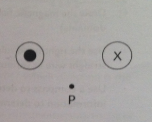# Problem: Two wires lie perpendicular to the plane of the paper, and equal electric currents pass through the paper in the directions shown. Point P is equidistant from the two wires.If the currents in both wires were instead directed out of the plane of the page (such that the current moved toward us), show the resultant magnetic field at point P.

###### FREE Expert Solution
94% (28 ratings)###### Problem DetailsTwo wires lie perpendicular to the plane of the paper, and equal electric currents pass through the paper in the directions shown. Point P is equidistant from the two wires.

If the currents in both wires were instead directed out of the plane of the page (such that the current moved toward us), show the resultant magnetic field at point P.